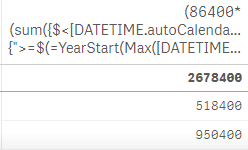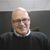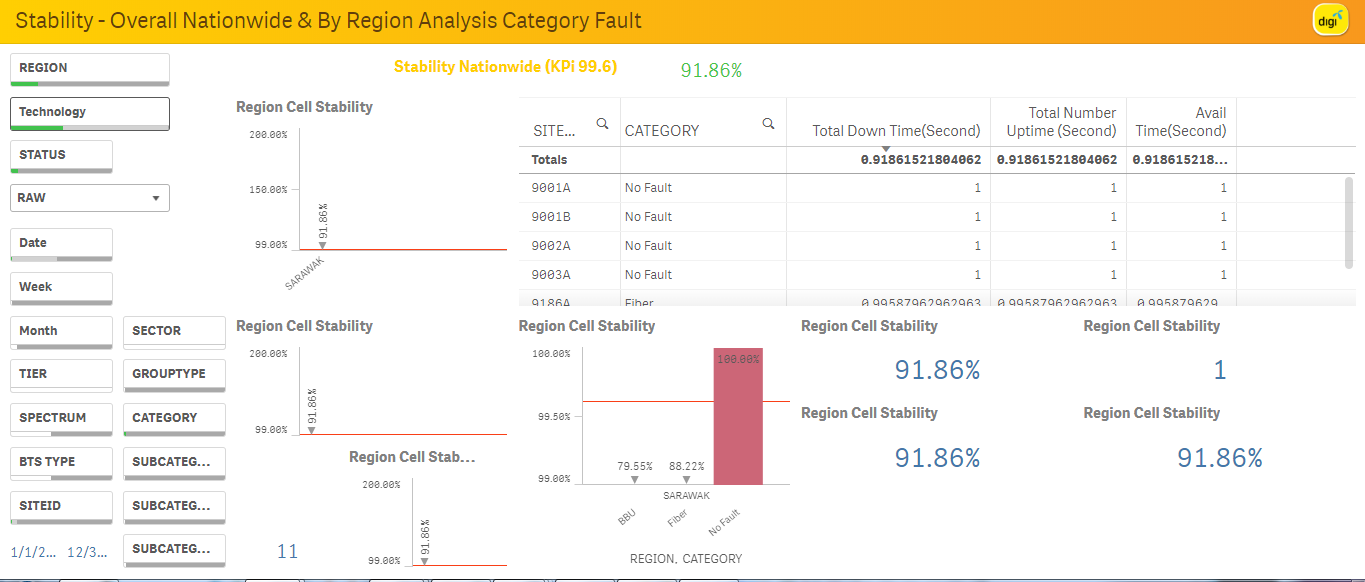# App Development

Announcements
Qlik Cloud Maintenance is scheduled between March 27-30. Visit Qlik Cloud Status page for more details.
cancel
Showing results for
Did you mean:Contributor III

## How to make a number static ?

Hi All,

Would like to ask how to make the number 2678400 static ?

The formula that was given to me is this :

(86400*(sum({\$<[DATETIME.autoCalendar.Date]={">=\$(=YearStart(Max([DATETIME.autoCalendar.Date]), 0))<=\$(=YearEnd(Max([DATETIME.autoCalendar.Date]), 0))"}>*<TECHNOLOGY = {'2G'}>} CELLNUMBER)))Regards,

Mahirah

6 RepliesPartner - Champion III

You have to explain what you mean by static ...

If you want to have it used multiple places you can put the calculation into a variable or a master measure.MVP

Static in the sense you don't want this number to change ...even when you make selection ?? in that case try like this

(86400*(sum({1<[DATETIME.autoCalendar.Date]={">=\$(=YearStart(Max([DATETIME.autoCalendar.Date]), 0))<=\$(=YearEnd(Max([DATETIME.autoCalendar.Date]), 0))"}>*<TECHNOLOGY = {'2G'}>} CELLNUMBER)))Contributor III
Author

By what I meant static is  :

if select any of the option on the left whether it is region , technology, category, etc , the number will stay static.

and I just want to use it to divide by a certain category.Contributor III
Author

Hi Avinash,

Seems like it doesn't work, everytime i clicked on any of the selection.Contributor II

Use the required formula in Variable and use afterwards where-ever required.MVP

Try like this

(86400*(sum({1<[DATETIME.autoCalendar.Date]={">=\$(=YearStart(Max({1}[DATETIME.autoCalendar.Date]), 0))<=\$(=YearEnd(Max({1}[DATETIME.autoCalendar.Date]), 0))"}>*<TECHNOLOGY = {'2G'}>} CELLNUMBER)))Tags
Community Browser